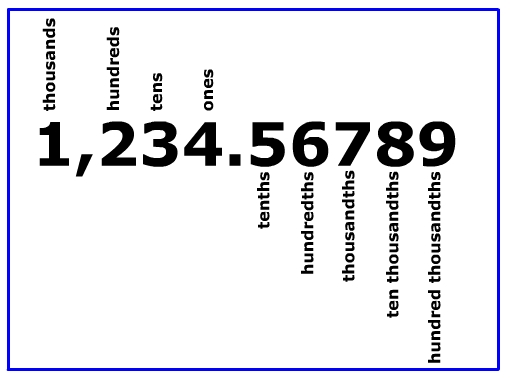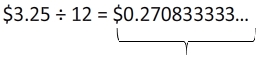# Rounding to the Nearest Hundredth

Have you ever seen the directions, round to the nearest cent?" These directions are actually asking you to round to the nearest hundredth.

Remember that the hundredths place is two moves from the right of the decimal point.To round to the nearest hundredth, we use the thousandths place to determine whether the hundredths place rounds up or stays the same.

Here are some examples.

1.) 0.3493
- Underline the hundredths place 0.3493
- Look to the right.  0.3493
If it is 5 or above, we give it a shove.
If it is 4 or below, we let it go.
- Round up to 0.35

2.) 15.921978
- Underline the hundredths place 15.921978
- Look to the right.  15.921975
If it is 5 or above, we give it a shove.
If it is 4 or below, we let it go.
- Round to 15.92

3.) 4,812.39812
- Underline the hundredths place4,812.39812
- Look to the right. 4,812.39812
If it is 5 or above, we give it a shove.
If it is 4 or below, we let it go.

In this example, it might be helpful to think of it as 39 rounding up to 40 instead of 9 rounding up to 10.

- Round to 4,812.40

Now let's take a look at some real life examples.

4.) A dozen eggs costs \$3.25. What is the cost of one egg?Notice that this answer doesnât look like money. So we need to round to the nearest penny, or the nearest hundredth.

0.2708333333.....

Notice that 7 is in the hundredths place and there is a 0 to the right. That tells us to keep the 7 the same and drop everything else to the right of it.

Each egg in the package costs about \$0.27.

5.) A package of socks cost \$8.48 for 6 pairs. What is the cost per pair of socks?

\$8.48 ÷ 6 = \$1.4133333333.....

Again, we have a number that doesn't look like an amount that would show up on a register at the store. So we will round to the nearest penny or the nearest hundredth.

1.41333333333.........

Notice that 1 is in the hundredths place and there is a 3 to the right. So

we will keep the 1 and round to \$1.41per pair of socks.

Let's Review:

To round to the nearest cent, nearest penny, or nearest hundredth, you will need to locate the hundredths place. Then look at the digit to the right. If it is 5 or above, the number in the hundredths place will be increased by 1 and all the rest of the numbers after it are dropped. If the number is 4 or below, you leave the digit in the hundredths place alone and drop all the rest of the numbers after.

Just remember, if the problem asks you to round to the nearest hundredth, make the end result look like money, meaning only two digits after the decimal point!